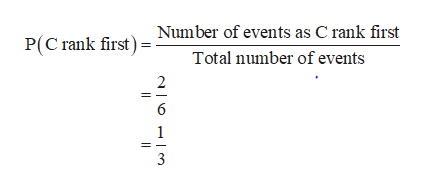# An individual is presented with three different glasses of cola, labeled C, D, and P. He is asked to taste all three and then list them in order of preference. Suppose the same cola has actually been put into all three glasses.a. What are the simple events in this ranking experiment, and what probability would you assign to each one?b. What is the probability that C is ranked first?c. What is the probability that C is ranked first and D is ranked last?

Question

An individual is presented with three different glasses of cola, labeled C, D, and P. He is asked to taste all three and then list them in order of preference. Suppose the same cola has actually been put into all three glasses.

a. What are the simple events in this ranking experiment, and what probability would you assign to each one?

b. What is the probability that C is ranked first?

c. What is the probability that C is ranked first and D is ranked last?

check_circle

Step 1

a. The simple events in this ranking experiment are {CDP, CPD, DCP, DPC, PCD, PDC}.

The probability assign to the first event is obtained as follows:

Step 2

This probability is same for all the six events. Thus, the probability assign to each event is 1/6.

Step 3

b. From the simple events it can be seen that simple events that C rank first is CDP and CPD. That is, the number of events that C ra...help_outlineImage TranscriptioncloseNumber of events as C rank first Total number of events P(C rank first) 2 6 1 3 fullscreen

### Want to see the full answer?

See Solution

#### Want to see this answer and more?

Solutions are written by subject experts who are available 24/7. Questions are typically answered within 1 hour.*

See Solution
*Response times may vary by subject and question.
Tagged in

### Probability